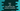# Get the only single element that satisfy a condition in dart list## Dart program to get an element from a list that satisfy a condition:

In this post, we will learn how to get an element from a list that satisfy a given condition. We can iterate over the items of a list and filter out the element that satisfy that condition.

Dart list also provides one method called singleWhere that finds one single element that satisfy a given condition.

In this post, we will learn how to use singleWhere to get an element from a list that satisfy a given con

### Definition:

singleWhere is defined as below:

``E singleWhere(bool testFunction(E e))``

This method takes one function testFunction and returns the first element that satisfy testFunction i.e. if testFunction returns true.

It works only if only element is there in the list that satisfies the condition.

### Error:

It throws StateError if there is no matching element or if there are multiple elements in the list.

### Example 1: Find the only even value from a list:

Let’s take a look at the below example:

``````void main() {
var givenList = [1, 2, 5, 7, 9, 101];

print(givenList.singleWhere((e) => e % 2 == 0));
}``````

This will print 2 as the output. Here, givenList is a list of numbers. The function inside print is using singleWhere in givenList to find out the only element which is even.

If we use a different list with more than one even elements like below,

``````void main() {
var givenList = [1, 2, 5, 7, 9, 101, 10];

print(givenList.singleWhere((e) => e % 2 == 0));
}``````

It will throw an error:

``Uncaught Error: Bad state: Too many elements``

### Example 2: singleWhere with custom objects:

Let’s use singleWhere with a list of custom objects. For the below example:

``````void main() {
var items = [
{"name": "Albert", "age": 20},
{"name": "Daisy", "age": 21},
{"name": "Noah", "age": 17}
];

print(items.singleWhere((e) => (e["age"] as double) % 2 == 0));
}``````

It will print:

``{name: Albert, age: 20}``